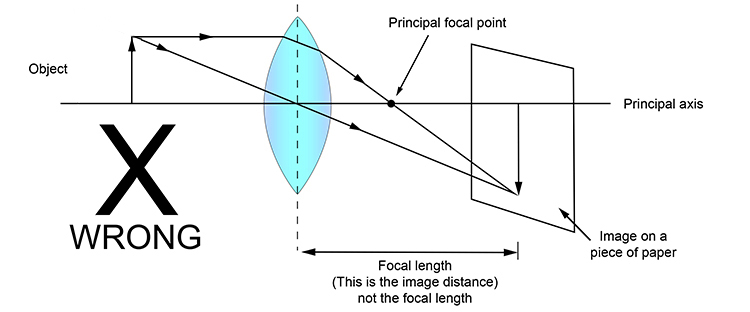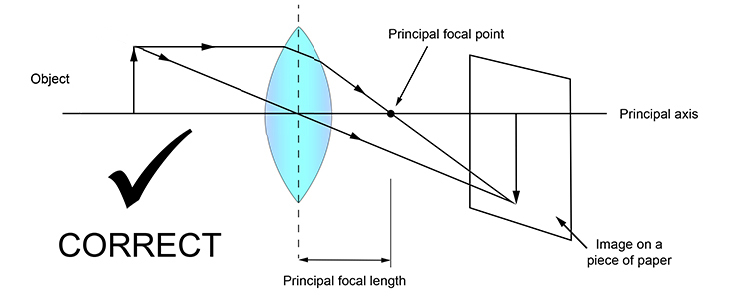# Principal focal length: be careful

To compare one convex lens with another, you need to know the focal length of each lens.

Some books define the focal length of a convex lens as the distance from the centre of the lens to where the image will appear focused on a piece of paper. But this is not correct: this is the "image distance", not the principal focal length.The focal length of a convex lens is the distance from the centre of the lens to the principal focal point and should always be called the principal focal length, as below:

NOTE: The line passing from the tip of the arrow through the centre point of the convex lens will be explained later.